# CBSEtips.in

## Saturday, 20 February 2021

### CBSE Class 9 Maths - MCQ and Online Tests - Unit 7 - Triangles

#### CBSE Class 9 Maths – MCQ and Online Tests – Unit 7 – Triangles

Every year CBSE  schools conducts Annual Assessment exams for 6,7,8,9,11th standards. These exams are very competitive to all the students. So our website provides online tests for all the 6,7,8,9,11th standard’s subjects. These tests are also very effective and useful for those who preparing for any competitive exams like Olympiad etc. It can boost their preparation level and confidence level by attempting these chapter wise online tests.

These online tests are based on latest CBSE syllabus. While attempting these our students can identify the weak lessons and continuously practice those lessons for attaining high marks. It also helps to revise the NCERT textbooks thoroughly.

#### CBSE Class 9 Maths – MCQ and Online Tests – Unit 7 – Triangles

Question 1.
In figure, D is the mid-point of side BC of a ?ABC and ?ABD = 50°. If AD = BD = CD, then find the measure of ?ACD.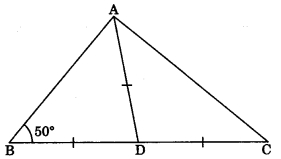(a) 30°
(b) 70°
(c) 80°
(d) 40°

Question 2.
In triangles ABC and PQR, AB = AC, ?C = ?P and ?B = ?Q. The two triangles are
(a) isosceles but not congruent
(b) isosceles and congruent
(c) congruent but not isosceles
(d) neither congruent nor isosceles

Answer: (a) isosceles but not congruent

Question 3.
In figure AB ? AE, BC ? AB, CE = DE and ?AED = 120°, then find ?ECD.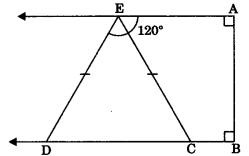(a) 80°
(b) 70°
(c) 85°
(d) 60°

Question 4.
In ?ABC, ?C = ?A and BC = 4 cm and AC = 5 cm, then find length of AB.
(a) 5 cm
(b) 3 cm
(c) 4 cm
(d) 2.5 cm

Question 5.
In ?ABC, AB = AC and ?B = 50°, then find ?C.
(a) 50°
(b) 40°
(c) 80°
(d) 120°

Question 6.
In figure if AE || DC and AB = AC, find the value of ?ABD.(a) 130°
(b) 110°
(c) 120°
(d) 70°

Question 7.
In figure X is a point in the interior of square ABCD, AXYZ is also a square. If DY = 3 cm and AZ = 2 cm, then find BY.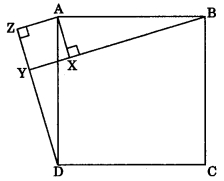(a) 5 cm
(b) 6 cm
(c) 7 cm
(d) 8 cm

Question 8.
In triangles ABC and DEF, AB = FD and ?A = ?D. The two triangles will be congruent by SAS axiom if
(a) BC = EF
(b) AC = DE
(c) AC = EF
(d) BC = DE

Question 9.
In given figure, the measure of ?BAC is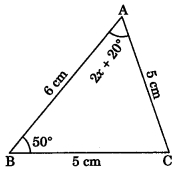(a) 60°
(b) 50°
(c) 70°
(d) 80°

Question 10.
In figure AB ? BE and EF ? BE. If BC = DE and AB = EF, then ?ABD is congruent to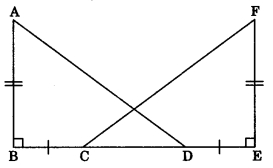(a) ?EFC
(b) ?ECF
(c) ?DEF
(d) ?FEC

Question 11.
D is a point on the side BC of a ?ABC such that AD bisects ?BAC. Then
(a) BD = CD
(b) BA > BD
(c) BD > BA
(d) CD > CA

Question 12.
It is given that ?ABC = ?FDE and AB = 5 cm, ?B = 40° and ?A = 80°. Then which of the following is true?
(?) DF = 5 cm, ?F = 60°
(b) DF = 5 cm, ?E = 60°
(c) DE = 5 cm, ?E = 60°
(d) DE = 5 cm, ?D = 40°Answer: (b) DF = 5 cm, ?E = 60°

Two triangles are congruent if their corresponding sides are equal in length and their corresponding angles are equal in size.
In the first option, DF = 5 cm but ?F is not equal to 60°.
In the third and the fourth options, the length of DE ? 5cm, since it is not defined.
In the second option, DF = 5cm and ?E = 60° is satisfied.

Question 13.
Two sides of a triangle are of lengths 5 cm and 1.5 cm. The length of the third side of the triangle cannot be
(a) 3.6 cm
(b) 4.1 cm
(c) 3.8 cm
(d) 3.4 cm

Question 14.
In ?PQR, if ?R > ?Q, then
(a) QR > PR
(b) PQ > PR
(c) PQ < PR
(d) QR < PR

Question 15.
In figure, ABC is an isosceles triangle whose side AC is produce to E and through C, CD is drawn parallel to BA. Find the value of x.(a) 52°
(b) 156°
(c) 76°
(d) 104°